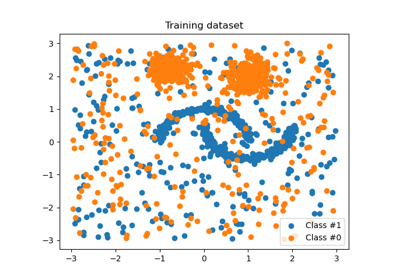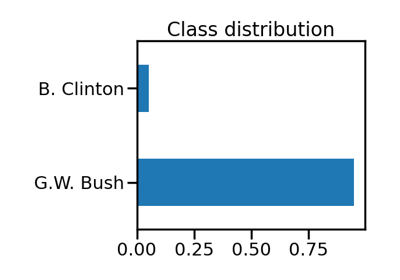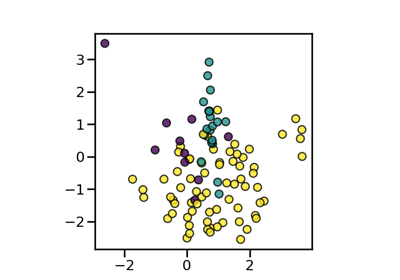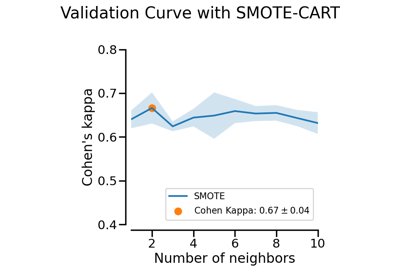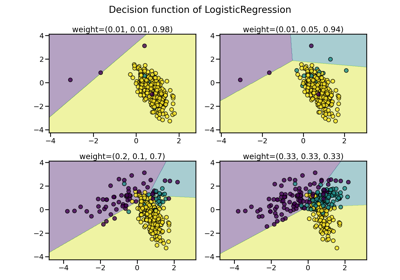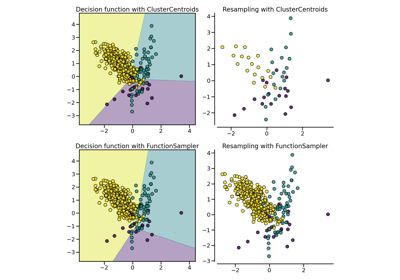# Pipeline¶

class imblearn.pipeline.Pipeline(steps, *, memory=None, verbose=False)[source]

Pipeline of transforms and resamples with a final estimator.

Sequentially apply a list of transforms, sampling, and a final estimator. Intermediate steps of the pipeline must be transformers or resamplers, that is, they must implement fit, transform and sample methods. The samplers are only applied during fit. The final estimator only needs to implement fit. The transformers and samplers in the pipeline can be cached using `memory` argument.

The purpose of the pipeline is to assemble several steps that can be cross-validated together while setting different parameters. For this, it enables setting parameters of the various steps using their names and the parameter name separated by a ‘__’, as in the example below. A step’s estimator may be replaced entirely by setting the parameter with its name to another estimator, or a transformer removed by setting it to ‘passthrough’ or `None`.

Parameters
stepslist

List of (name, transform) tuples (implementing fit/transform/fit_resample) that are chained, in the order in which they are chained, with the last object an estimator.

memoryInstance of joblib.Memory or str, default=None

Used to cache the fitted transformers of the pipeline. By default, no caching is performed. If a string is given, it is the path to the caching directory. Enabling caching triggers a clone of the transformers before fitting. Therefore, the transformer instance given to the pipeline cannot be inspected directly. Use the attribute `named_steps` or `steps` to inspect estimators within the pipeline. Caching the transformers is advantageous when fitting is time consuming.

verbosebool, default=False

If True, the time elapsed while fitting each step will be printed as it is completed.

Attributes
named_steps`Bunch`

Read-only attribute to access any step parameter by user given name. Keys are step names and values are steps parameters.

`make_pipeline`

Helper function to make pipeline.

Notes

Examples

```>>> from collections import Counter
>>> from sklearn.datasets import make_classification
>>> from sklearn.model_selection import train_test_split as tts
>>> from sklearn.decomposition import PCA
>>> from sklearn.neighbors import KNeighborsClassifier as KNN
>>> from sklearn.metrics import classification_report
>>> from imblearn.over_sampling import SMOTE
>>> from imblearn.pipeline import Pipeline
>>> X, y = make_classification(n_classes=2, class_sep=2,
... weights=[0.1, 0.9], n_informative=3, n_redundant=1, flip_y=0,
... n_features=20, n_clusters_per_class=1, n_samples=1000, random_state=10)
>>> print(f'Original dataset shape {Counter(y)}')
Original dataset shape Counter({1: 900, 0: 100})
>>> pca = PCA()
>>> smt = SMOTE(random_state=42)
>>> knn = KNN()
>>> pipeline = Pipeline([('smt', smt), ('pca', pca), ('knn', knn)])
>>> X_train, X_test, y_train, y_test = tts(X, y, random_state=42)
>>> pipeline.fit(X_train, y_train)
Pipeline(...)
>>> y_hat = pipeline.predict(X_test)
>>> print(classification_report(y_test, y_hat))
precision    recall  f1-score   support

0       0.87      1.00      0.93        26
1       1.00      0.98      0.99       224

accuracy                           0.98       250
macro avg       0.93      0.99      0.96       250
weighted avg       0.99      0.98      0.98       250
```

Methods

 Apply transforms, and decision_function of the final estimator `fit`(X[, y]) Fit the model. `fit_predict`(X[, y]) Apply `fit_predict` of last step in pipeline after transforms. `fit_resample`(X[, y]) Fit the model and sample with the final estimator. `fit_transform`(X[, y]) Fit the model and transform with the final estimator. `get_params`([deep]) Get parameters for this estimator. `predict`(X, **predict_params) Apply transforms to the data, and predict with the final estimator Apply transforms, and predict_log_proba of the final estimator Apply transforms, and predict_proba of the final estimator `score`(X[, y, sample_weight]) Apply transforms, and score with the final estimator Apply transforms, and score_samples of the final estimator. `set_params`(**kwargs) Set the parameters of this estimator.
decision_function(X)[source]

Apply transforms, and decision_function of the final estimator

Parameters
Xiterable

Data to predict on. Must fulfill input requirements of first step of the pipeline.

Returns
y_scorearray-like of shape (n_samples, n_classes)
fit(X, y=None, **fit_params)[source]

Fit the model.

Fit all the transforms/samplers one after the other and transform/sample the data, then fit the transformed/sampled data using the final estimator.

Parameters
Xiterable

Training data. Must fulfill input requirements of first step of the pipeline.

yiterable, default=None

Training targets. Must fulfill label requirements for all steps of the pipeline.

**fit_paramsdict of str -> object

Parameters passed to the `fit` method of each step, where each parameter name is prefixed such that parameter `p` for step `s` has key `s__p`.

Returns
selfPipeline

This estimator.

fit_predict(X, y=None, **fit_params)[source]

Apply `fit_predict` of last step in pipeline after transforms.

Applies fit_transforms of a pipeline to the data, followed by the fit_predict method of the final estimator in the pipeline. Valid only if the final estimator implements fit_predict.

Parameters
Xiterable

Training data. Must fulfill input requirements of first step of the pipeline.

yiterable, default=None

Training targets. Must fulfill label requirements for all steps of the pipeline.

**fit_paramsdict of string -> object

Parameters passed to the `fit` method of each step, where each parameter name is prefixed such that parameter `p` for step `s` has key `s__p`.

Returns
y_predndarray of shape (n_samples,)

The predicted target.

fit_resample(X, y=None, **fit_params)[source]

Fit the model and sample with the final estimator.

Fits all the transformers/samplers one after the other and transform/sample the data, then uses fit_resample on transformed data with the final estimator.

Parameters
Xiterable

Training data. Must fulfill input requirements of first step of the pipeline.

yiterable, default=None

Training targets. Must fulfill label requirements for all steps of the pipeline.

**fit_paramsdict of string -> object

Parameters passed to the `fit` method of each step, where each parameter name is prefixed such that parameter `p` for step `s` has key `s__p`.

Returns
Xtarray-like of shape (n_samples, n_transformed_features)

Transformed samples.

ytarray-like of shape (n_samples, n_transformed_features)

Transformed target.

fit_transform(X, y=None, **fit_params)[source]

Fit the model and transform with the final estimator.

Fits all the transformers/samplers one after the other and transform/sample the data, then uses fit_transform on transformed data with the final estimator.

Parameters
Xiterable

Training data. Must fulfill input requirements of first step of the pipeline.

yiterable, default=None

Training targets. Must fulfill label requirements for all steps of the pipeline.

**fit_paramsdict of string -> object

Parameters passed to the `fit` method of each step, where each parameter name is prefixed such that parameter `p` for step `s` has key `s__p`.

Returns
Xtarray-like of shape (n_samples, n_transformed_features)

Transformed samples.

get_params(deep=True)[source]

Get parameters for this estimator.

Returns the parameters given in the constructor as well as the estimators contained within the `steps` of the `Pipeline`.

Parameters
deepbool, default=True

If True, will return the parameters for this estimator and contained subobjects that are estimators.

Returns
paramsmapping of string to any

Parameter names mapped to their values.

property inverse_transform

Apply inverse transformations in reverse order

All estimators in the pipeline must support `inverse_transform`.

Parameters
Xtarray-like of shape (n_samples, n_transformed_features)

Data samples, where `n_samples` is the number of samples and `n_features` is the number of features. Must fulfill input requirements of last step of pipeline’s `inverse_transform` method.

Returns
Xtarray-like of shape (n_samples, n_features)
predict(X, **predict_params)[source]

Apply transforms to the data, and predict with the final estimator

Parameters
Xiterable

Data to predict on. Must fulfill input requirements of first step of the pipeline.

**predict_paramsdict of string -> object

Parameters to the `predict` called at the end of all transformations in the pipeline. Note that while this may be used to return uncertainties from some models with return_std or return_cov, uncertainties that are generated by the transformations in the pipeline are not propagated to the final estimator.

New in version 0.20.

Returns
y_predarray-like
predict_log_proba(X)[source]

Apply transforms, and predict_log_proba of the final estimator

Parameters
Xiterable

Data to predict on. Must fulfill input requirements of first step of the pipeline.

Returns
y_scorearray-like of shape (n_samples, n_classes)
predict_proba(X)[source]

Apply transforms, and predict_proba of the final estimator

Parameters
Xiterable

Data to predict on. Must fulfill input requirements of first step of the pipeline.

Returns
y_probaarray-like of shape (n_samples, n_classes)
score(X, y=None, sample_weight=None)[source]

Apply transforms, and score with the final estimator

Parameters
Xiterable

Data to predict on. Must fulfill input requirements of first step of the pipeline.

yiterable, default=None

Targets used for scoring. Must fulfill label requirements for all steps of the pipeline.

sample_weightarray-like, default=None

If not None, this argument is passed as `sample_weight` keyword argument to the `score` method of the final estimator.

Returns
scorefloat
score_samples(X)[source]

Apply transforms, and score_samples of the final estimator.

Parameters
Xiterable

Data to predict on. Must fulfill input requirements of first step of the pipeline.

Returns
y_scorendarray of shape (n_samples,)
set_params(**kwargs)[source]

Set the parameters of this estimator.

Valid parameter keys can be listed with `get_params()`. Note that you can directly set the parameters of the estimators contained in `steps`.

Returns
self
property transform

Apply transforms, and transform with the final estimator

This also works where final estimator is `None`: all prior transformations are applied.

Parameters
Xiterable

Data to transform. Must fulfill input requirements of first step of the pipeline.

Returns
Xtarray-like of shape (n_samples, n_transformed_features)

## Examples using `imblearn.pipeline.Pipeline`¶